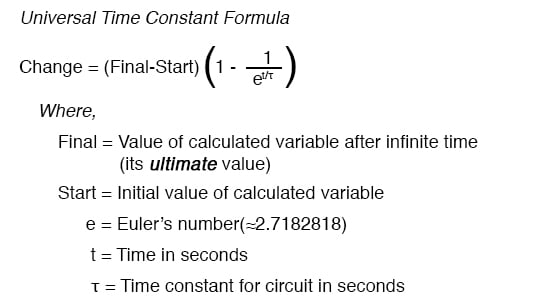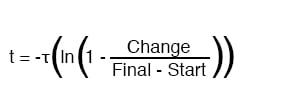Vol. EE Reference
Chapter 1 Useful Equations And Conversion Factors

# Time Constant Equations

### Value of time constant in series RC and RL circuits

Time constant in seconds = RC

Time constant in seconds = L/R

### Calculating voltage or current at specified time### Calculating time at specified voltage or currentRELATED WORKSHEETS:

#### Lessons in Electric Circuits

##### Volumes »
Published under the terms and conditions of the Design Science License